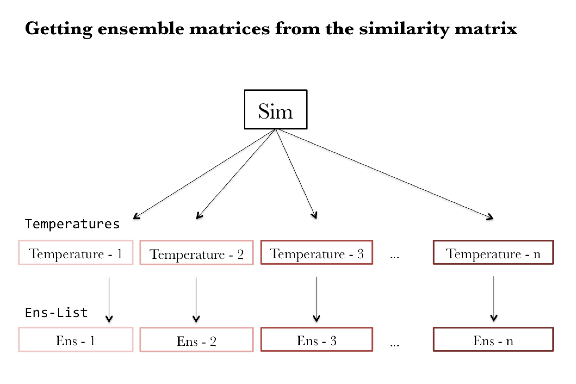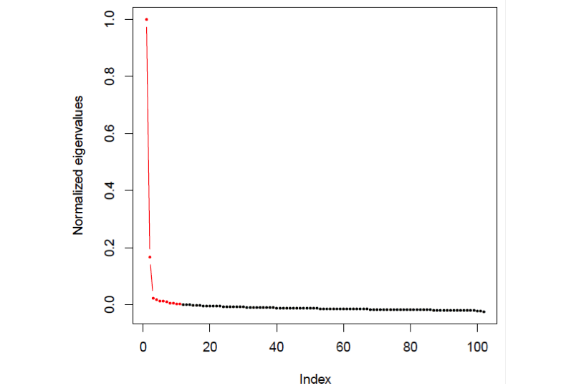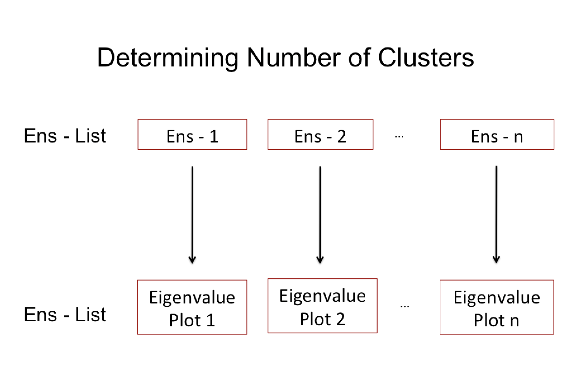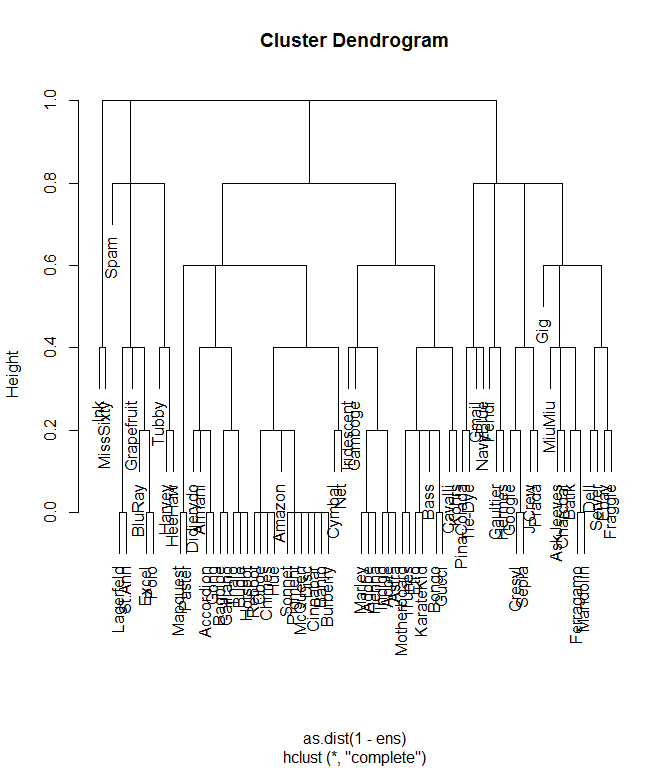# Introduction to DCG

#### Introduction

The R code for data cloud geometry is designed to find multi-level structure in undirected network data (e.g. edgelist or matrix)[Chen and Fushing 2012]. This multi-level structure is equivalent to finding community structure, similar to the Girvan-Newman method of finding network modularity [Newman and Girvan 2004]. This method begins with a similarity matrix, where the entries of the matrix are a measure of how similar a pair of individuals is with respect to a particular feature. Similarity can be measured based on grooming relationships (where the strength of grooming per dyad is the measure of similarity) or other affiliative relationships. Similarity can also be measured using physical traits or genetic code. The data cloud geometry method involves performing a random walk across all nodes in the network. The probability of making a step from one node to another is based upon their strength of similarity (i.e. from the value in the similarity matrix). Such random walks proceed locally, going to and from a local subset of nodes. Once a subset of nodes has been visited sufficiently (e.g. when one node from that community has been visited at least 5 times, m = 5) that node is removed from the network such that it may no longer be visited. Once all nodes from a community have been removed in this manner, the random walk is forced to jump to another community. This is how communities are defined – the subset of nodes that is each visited 5 times before jumping to a new set of nodes is one community.

#### Importing data

DCG package works on similarity matrices. It required the raw data to be an edgelist or a matrix. DCG is designed for undirected networks, i.e. where the direction of the interaction doesn’t matter. Therefore, we use as.symmetricAdjacencyMatrix to convert the raw data (an edgelist or a matrix) to a symmetric adjacency matrix. as.SimilarityMatrix further converts the symmetric adjacency matrix to a similarity matrix whose values range from 0 to 1 by dividing values in the raw matrix by the maximum value of the matrix. You could assess help for all functions by running ?functionName, for example, ?as.symmetricAdjacencyMatrix.

library(DCG)
head(monkeyGrooming)
##   Initiator Recipient Groom
## 1 Accordion     Astra     1
## 2 Accordion     Banjo     3
## 3 Accordion    Chimes     1
## 4 Accordion  Cinnabar     2
## 5 Accordion    Cymbal     7
## 6 Accordion   Pigment     3
symmetricAdjacencyMatrix <- as.symmetricAdjacencyMatrix(monkeyGrooming, weighted = TRUE, rule = "weak")

Sim <- as.SimilarityMatrix(symmetricAdjacencyMatrix)

#### Selecting temperatures

Because the random walk proceeds based upon similarity, the range of variance in similarity will influence how close two nodes appear to be. Therefore, the similarity matrix must be evaluated at several different ‘temperatures’. For example, the similarity matrix Sim can be transformed to Sim^0.5, Sim^2, Sim^5, and Sim^10; the scale of variance within each matrix will allow the random walk to assess similarity at different levels.

To generate several different temperatures, we use the function temperatureSample with arguments start and end which define the lowest and highest temperatuers respectively. The argument n defined the number of temperatures you want to sample, with default being 20. It is recommended to use at least 20-30 different temperatures. Because the number of distinct clusters in the network may be different at each temperature, and these values can be plotted to note which cluster numbers hold stable for multiple temperatures (meaning this number is likely a logical division of the network) and which cluster numbers are ephemeral. The method argument of temperatureSample allows you to choose how you want to sample the temperatures: randomly ("random") or based on fixed intervals ("fixedInterval").

set.seed(18) # This ensure we get the same results when sampling temperares. It is not required.

temperatures <- temperatureSample(start = 0.01, end = 10, n = 30, method = 'fixedInterval') 

#### Generating ensemble matrices

The function getEnsList involves two steps. It first transforms the similarity matrix Sim into a list of matrices of different scaled variances based on temperatures. Then it performed n-times of consecutive regulated random walks (defined by MaxIt, MaxIt = 1000 by default) with the node removal parameter m (m = 5 by default) on each matrix in the list. It returned a list of ensemble matrices which is used later on in determining clusters. We used MaxIt = 5 for illustration purpose only because it ran quickly.

Ens_list <- getEnsList(simMat = Sim, temperatures = temperatures, MaxIt = 5, m = 5)

The process of transforming similarity matrix into a list of ensemble matrices is illustrated in the following flow chart.#### Generating eigenvalue plots

The appropriate number of clusters is determined by examining the eigenvalue plots. The function plotMultiEigenvalues is used to plot eigenvalues corresponding to each ensemble matrix in the list of ensemble matrix (Ens_List). These plots show the approximate number of clusters. Red dots connected by a red line represent a cluster. The plot below shows two red lines that connect three red dots on the left, which indicates the presence of two clusters.The function plotMultiEigenvalues takes two arguments, Enslist and mfrow, and generate eigenvalue plots which allow you to use elbow rules to decide number of communities present in your network. The argument Enslist is the output from the function getEnsList. There is one ensemble matrix corresponding to each temperature. The number of ensemble matrices will be the same as the number of temperatures you specified when generating the temperatures. The second argument mfrow specifies the arrangement of the eigenvalue plots. The function mfrow takes two values in the format of c(integer_row_number, integer_column_number). For example, if you have 20 ensemble matrices, and you want generate a graph of 2 plots in each row and 10 plots in each column, you could specify mfrow = c(10, 2). In addition, the function plotMultiEigenvalues also take other arguments that are in plot. You could specify font size, title, etc by accessing arguments in plot. It is recommended that you plot into a pdf device, instead of in R console. Plotting into a pdf device allows a better view.

# start a pdf graphic device
pdf(file = "./myeigenvalueplots.pdf", onefile = TRUE, width = 20, height = 40)
# plot in the pdf device you started
plotMultiEigenvalues(Ens_list, mfrow = c(10, 2),
mar = c(1, 1, 1, 1),
line = -1.5, cex = 0.8)
graphics.off() # close all plotting devices.
# You could find you plots in your working directory: "./myeigenvalueplots.pdf"

Note, the plots in your “eigenvalue_plots.pdf” file look messy because we ran only 5 iterations (MaxIt = 5) in getEnsList. Try using MaxIt = 1000 and see if your plots are of any difference.

The process generating eigenvalue plots from ensemble matrices is shown in the flow chart below.After inspecting the eigen plots, choose an ensemble matrix (Ens1, Ens2….Ens20) whose number of clusters appears to be stable across multiple temperatures. For example, if matrices Ens1 – Ens4 all show two clusters, Ens5 shows three clusters, and Ens6 – Ens20 all show four clusters, then the divisions of two or four clusters are likely reasonable divisions of your network. Once you know which ensemble matrix to choose, you’ll need to generate tree plots to examine clusters.

#### Generating tree plots

To generate tree plot of specific ensemble matrix, you could use plotTheCluster function. For example, if you want to create the tree plot from only the first ensemble matrix, you’ll need to run the following codes:

plotTheCluster(Ens_list, 2)The function plotCLUSTERS is used to plot tree plots from a list of ensemble matrices. Its first argument EnsList is the output from the function getEnsList. The plotting mechanism works similiar to plotMultiEigenvalues. Again, because we are plotting multiple plots at the same time, it is better to export these plots to a pdf file so that you could see each of the plot clearly. You could find the exported .pdf file in your working directory.

pdf(file = "./ClustersPlot.pdf", width = 20, height = 60)

plotCLUSTERS(EnsList = Ens_list,
mfrow = c(10, 2),
mar = c(1,1,1,1),
line = -1.5,
cex = 0.8)

dev.off()

In addition, the Ens matices contains valuable information as well. These values range from 0 to 1 and represent the proportion of the 1000 iterations in which each pair of nodes appeared in the same cluster.

To examine ensemble matrices of your choice, you could pull the matrix from the list by running Ens_list[[n]], with n being the index of the matrix you choose. For example, if you want to examine the first one, simply run Ens_list[]. If you want to save the first matrix into an Excel file, run write.csv(Ens_list[], "myFirstEnsembleMatrix.csv").